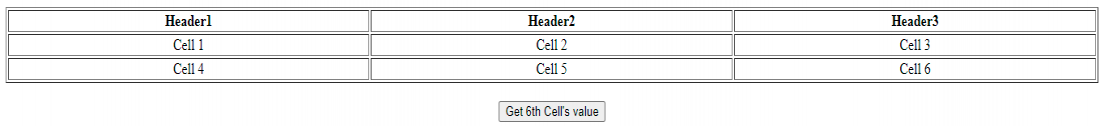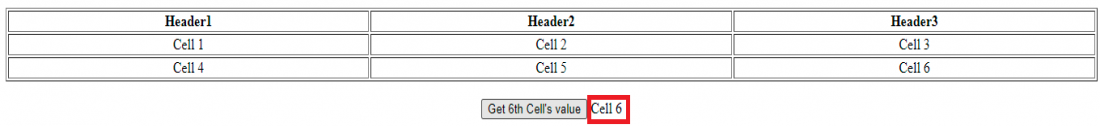Open in App
Not now

# How to find the sixth cell (second row and third column ) of a 3×3 table in jQuery ?

• Last Updated : 18 Apr, 2021

In this article, we will see how to get the sixth cell of a 3×3 table in jQuery. To find the nth-child of an element, we can use the nth-child selector of jQuery.

Approach: Sixth cell of a 3×3 table can be found using the following jQuery call:

`\$('#table1 tr:nth-child(2) td:nth-child(3)').text();`

If the table has column headers, the sixth cell can be found using the following call.

`\$('#table1 tr:nth-child(3) td:nth-child(3)').text();`

HTML code: Please note that the nth-child selector’s index is 1 based.

## HTML

 ```<``html``>`` ` `<``head``>``    ``<``script` `type``=``"text/javascript"` `src``=``"https://code.jquery.com/jquery-3.6.0.min.js"``>``    ```` ` `    ``<``script` `type``=``"text/javascript"``>``        ``\$(document).ready(function () {``            ``\$('#btnGetValue').click(function () {``                ``\$('#value').text(\$(``'#table1 tr:nth-child(3) td:nth-child(3)').text());``            ``});``        ``});``    `````` ` `<``body` `style``=``"text-align:center"``>``    ``<``table` `id``=``"table1"` `style``=``        ``"width:100%"` `border``=``"1"``>``        ``<``tr``>``            ``<``th``>``                ``Header1``            ````            ``<``th``>``                ``Header2``            ````            ``<``th``>``                ``Header3``            ````        ````        ``<``tr``>``            ``<``td``>Cell 1``            ``<``td``>Cell 2``            ``<``td``>Cell 3``        ````        ``<``tr``>``            ``<``td``>Cell 4``            ``<``td``>Cell 5``            ``<``td``>Cell 6``        ````    ````    ``<``br``>`` ` `    ``<``input` `type``=``"button"` `        ``value``=``"Get 6th Cell's value"` `        ``id``=``"btnGetValue"` `/>``         ` `    ``<``span` `id``=``"value"``>```` ` ``

Output: We see the following web page.

• Before Click:• After Click:

You see the sixth cell’s value next to the button (highlighted in red rectangle)After click output

My Personal Notes arrow_drop_up